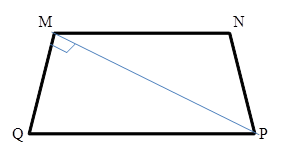Chapter 4.4, Problem 21EElementary Geometry For College St...

7th Edition
Alexander + 2 others
ISBN: 9781337614085

Solutions

Chapter
SectionElementary Geometry For College St...

7th Edition
Alexander + 2 others
ISBN: 9781337614085
Textbook Problem

In isosceles trapezoid MNPQ with M N ¯ | | Q P ¯ , diagonal M P ¯ ⊥ M Q ¯ . If PQ = 13 and NP = 5, how long is diagonal M P ¯ ?To determine

To Find:

The length of the diagonal MP¯ of the trapezoid MNPQ.

Explanation

Consider the following isosceles trapezoid.

Given: PQ = 13 and NP = 5.

Also, MN¯||QP¯ and the diagonal MP¯MQ¯.

We know that for isosceles trapezoid NPMQ.

Therefore, MQ = 5.

Consider the ΔMPQ

Still sussing out bartleby?

Check out a sample textbook solution.

See a sample solution

The Solution to Your Study Problems

Bartleby provides explanations to thousands of textbook problems written by our experts, many with advanced degrees!

Get Started

Find more solutions based on key concepts